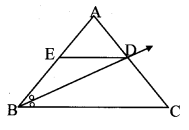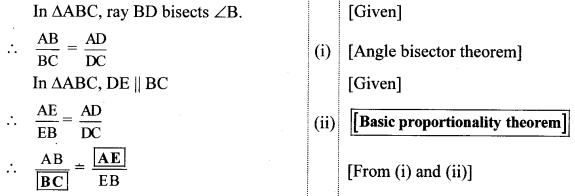# Maharashtra Board Class 10 Maths-2 Solutions Chapter 1 Similarity Practice Set 1.2

## Maharashtra Board Class 10 Maths-2 Solutions Chapter 1 Similarity Practice Set 1.2

### Part-2

Question 2.
Write another proof of the above theorem (property of an angle bisector of a triangle). Use the following properties and write the proof.
i. The areas of two triangles of equal height are proportional to their bases.
ii. Every point on the bisector of an angle is equidistant from the sides of the angle. (Textbook pg. no. 9)Given: In ∆CAB, ray AD bisects ∠A.
To prove: ABAC = BDDC
Construction: Draw seg DM ⊥ seg AB A – M – B and seg DN ⊥ seg AC, A – N – C.
Solution:
Proof:
In ∆ABC,
Point D is on angle bisector of ∠A. [Given]
∴DM = DN [Every point on the bisector of an angle is equidistant from the sides of the angle]
A(ΔABD)A(ΔACD)=AB×DMAC×DN [Ratio of areas of two triangles is equal to the ratio of the product of their bases and corresponding heights]
∴ A(ΔABD)A(ΔACD)=ABAC (ii) [From (i)]
Also, ∆ABD and ∆ACD have equal height.
∴ A(ΔABD)A(ΔACD)=BDCD (iii) [Triangles having equal height]
ABAC=BDDC [From (ii) and (iii)]

Question 3.
i. Draw three parallel lines.
ii. Label them as l, m, n.
iii. Draw transversals t1 and t2.
iv. AB and BC are intercepts on transversal t1.
v. PQ and QR are intercepts on transversal t2.
vi. Find ratios ABBC and PQQR. You will find that they are almost equal. Verify that they are equal.(Textbook pg, no. 10)
Solution:(Students should draw figures similar to the ones given and verify the properties.)

Question 4.
In the adjoining figure, AB || CD || EF. If AC = 5.4, CE = 9, BD = 7.5, then find DF.(Textbook pg, no. 12)Solution:Question 5.
In ∆ABC, ray BD bisects ∠ABC. A – D – C, side DE || side BC, A – E – B, then prove that ABBC = AEEB (Textbook pg, no. 13)Solution: